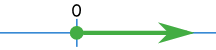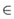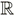# 函数复合

"函数复合"是把一个函数的输出作为另一个函数的输入：f() 的结果代入 g()

### 例子：f(x) = 2x+3，g(x) = x2

"x" 只是个位置标志符，为简单起见，在这里我们称之为 "输入"：

f(输入) = 2(输入)+3

g(输入) = (输入)2

(g º f)(x) = g(f(x))(g º f)(x) = (2x+3)2

(f º g)(x) = f(g(x))(f º g)(x) = 2x2+3

(g º f)(x)

## 与自己复合

### 例子：f(x) = 2x+3

(f º f)(x) = f(f(x))(f º f)(x) = 2(2x+3)+3 = 4x + 9

 (f º f)(x) = f(f(x)) = f(2x+3) = 2(2x+3)+3 = 4x + 9

## 定义域### 例子：√x（x 的平方根）的定义域

√x 的定义域是所有非负实数{ x| x ≥ 0}

[0,+∞)

## 复合函数的定义域

• f(x) 的定义域要正确，
• g(x) 的定义域也要正确

### 例子：f(x) = √x and g(x) = x2

f(x) = √x 的定义域是所有非负实数

g(x) = x2 的定义域是所有实数

 (g º f)(x) = g(f(x)) = (√x)2 = x

…… 但因为在这里它是个复合函数，所以我们也要考虑 f(x) 的定义域

## 为什么要考虑两个定义域？你看到最后结果是的钻出来的洞，所以你可能以为这个复合机器用在木头和金属上都可以。 但是，如果你把木头放进 g º f 这个复合机器里，第一个函数 f 就会把木头烧掉！

## 分解函数

### 例子： (x+1/x)2

f(x) = x + 1/x

g(x) = x2

 (g º f)(x) = g(f(x)) = g(x + 1/x) = (x + 1/x)2

## 总括

• "函数复合" 是把一个函数的输出作为另一个函数的输入。
• (g º f)(x) = g(f(x)), 先运用 f()，然后运用 g()
• 一定要符合第一个函数的定义域
• 有一些函数可以被分解成两个或以上比较简单的函数。